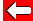Quadratic equations take the following form:
ax² + bx + c = 0

Where x is the only variable and a, b and c are just numbers (constants, that may also be zero!)

If a=0 then the equation is not quadratic: bx + c = 0

However, if b=0 then it can be: ax² + c = 0

Whilst if c=0 then it's: ax² + bx = 0

It is all much less confusing with numbers!Go back a pageMaths MenuGo to next page